# Bridging the gap: The Boltzmann Distribution, traditional science and machine learning

A story of entropy as told through a probability distribution

## Introduction

The fields of chemistry and physics are not truly distinct, and knowledge from one often informs the other. There are few places where this is more apparent than when discussing topics in quantum mechanics and thermodynamics. As anyone who has taken more than one course in these will tell you, beyond a point they begin to blend together. But nevertheless, making a distinction between them can be helpful.

How can we do that? Let’s begin with a container of air. And then zoom into a single molecule of water vapour inside it.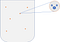Fig. 1. A schematic for a closed container full of air with one water vapour molecule highlighted

How does this molecule behave? It is not enough to say electrons are being shared to form chemical bonds. How are they being shared? (Hint). How do these electrons behave? (Hint). What happens if this molecule is struck by light? (Hint). These are all questions that can be answered by quantum mechanics.

Let’s zoom out a little to a scale we can perceive more easily. What is the temperature of this container? What happens to the pressure inside this closed container if we start to heat it up? (Hint). If we supply the container with heat, what happens to the speed of molecules in the container? (Hint). These feel like questions with more instinctive answers. But to be answered robustly, we turn to thermodynamics.

So now we know what ideas describe this container at micro and macro scale. What happens during the transition between the two? Let’s illustrate that with a question:

Why don’t the gas molecules settle down in a corner of the container? Why do they expand to fill the volume of the container?

Answer: Because the entropy of this gas increases as the thermal energy of the molecules spreads out into a larger space.

An isolated system spontaneously moves towards thermodynamic equilibrium, which is the state with maximum entropy. And entropy spans the difference between quantum mechanics and thermodynamics, forming the basis of statistical mechanics. Entropy is what describes the randomness of a system from micro to macro scale. Entropy is what makes our universe expand and explains all life in it. Entropy makes the universe fascinating. Entropy will lead to the death of the Universe.

## Understanding Entropy

So let’s talk a little bit about entropy. The textbook definition of entropy the first time students encounter it is usually “a measure of the randomness of a system”. But now that I’ve told you that the idea of entropy spans the scale of quantum to universe, you can appreciate the idea that “randomness” means something different at micro and macro scales.

In classical thermodynamics, entropy(measured in joules/kelvin) was first described in the early 1850s by the Clausius inequality. It arose as a way to explain the second law of thermodynamics: “The second law of thermodynamics states that the total entropy of an isolated system can never decrease over time, and is constant if and only if all processes are reversible.” In layman’s terms, the universe wants to increase entropy, and to increase entropy is to increase randomness.

Without getting too caught up in specifics, we examine the Clausius inequality: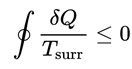Fig. 2. Clausius inequality, where the numerator describes an infinitesimal amount of heat absorbed absorbed by the system from the surroundings, while the denominator is the temperature of the surroundings. The closed integral is carried out along a thermodynamic process path (essentially the path on a P-V or similar 2D graph that explains a thermodynamic process).

This does not seem to have anything to do with randomness or entropy. But suspend your disbelief and think about the consequences of this inequality. All Clausius was trying to say was that it is impossible to construct a device whose sole effect is to transfer heat from a colder reservoir to a hotter reservoir. Heat wants to flow from hot to cold, and if we want to construct a device that does the opposite, we must provide entropy to the system. This is a simplified way of explaining a longer derivation, and the incredible end result can be described through the following inequality: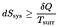Fig. 3. Simplified entropy equation for classical thermodynamics. The condition for = is when the processes are reversible.

Amazingly, entropy is one of the few situations in science where a macro scale idea is more difficult to grasp than one at micro scale. The Gibbs entropy formula quantifies the entropy for (comparatively) micro-scale particles.

We start with a simple statement: “The macroscopic state of a system is characterized by a distribution of its microstates.” Here, a microstate is a specific microscopic configuration a system can have (think of the way the molecules arranged themselves in our initial container of air example). A system fluctuates between these configurations constantly.

Then, if a microstate i has energy E_i, and p_i is the probability that this microstate occurs during the system’s fluctuations, the entropy of the system can be described as: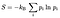Fig. 4. Gibbs formula for entropy

Here, the constant kB is Boltzmann’s constant, with units joules/kelvin.

We started this discussion with the idea that quantum/statistical mechanics and thermodynamics find equivalency in some topics. Now you’ve seen how entropy is quantified at macro and micro scales. But they don’t look similar at all. For starters, the macro scale formula involves heat and temperature, while the micro scale formula deals with microstates and their configurations. How do we bridge the gap?

## The Boltzmann Distribution

Our aim to bridge the gap between the two definitions of entropy starts with a question: Does the energy of a configuration influence the probability that the system exists in that configuration? Turns out, it does.

This concept is illustrated by the Boltzmann distribution, a probability distribution that gives the probability of a system existing in a certain state as a function of that state’s energy and the temperature of the system being studied.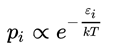Fig. 5. Boltzmann distribution, p_i is the probability of the system being in state i, epsilon_i is the energy of that state, k is Boltzmann’s constant, and T is the temperature of the system.

What does “system” refer to here? Well, keeping in mind that we are bridging the gap between quantum mechanics and thermodynamics, “system” can be anything from a single atom to a planetary body. The beauty of the Boltzmann distribution is that in a short relationship of proportionality it captures a fundamental truth about the universe: A state with lower energy has higher probability of being occupied.

We can actually spell the distribution out a little more like typical mathematical distributions: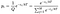Fig. 6. The Boltzmann distribution (all variables mean the same as in Fig. 5 with the addition of M, which is the number of all states accessible to the system being studied)

As is clear, this is a further resolved version of the Boltzmann distribution from Fig. 5, with the proportionality symbol resolved into a proportionality constant Q (Note: This is not the same as the Q from our thermodynamic entropy equation).

This Q is an important concept in physics — the canonical partition function.

On first glance there might appear to be nothing special about it. It stems from a simple mathematical idea: The sum of probabilities for all states that a system can access has to be 1. “Well naturally,” one might say, “Probabilities for an exhaustive list of outputs should add up to 1.” That seems obvious to you now, but what of physicists and chemists who had to walk through each step of that idea painstakingly, because they could assume nothing about it? Why wouldn’t there be an infinite number of states a random system can occupy? The idea that there is an exhaustive list of states that a system can access and we can in fact calculate the energy levels for each of those states thus building a partition function is nothing short of revolutionary.

Think about an atom. Think of how difficult it is to conceptualize anything about something that small. Now try to imagine that there is a numbered finite list of ways that this atom can exist and each of those ways has a certain specific energy level that can be calculated. In fact, each of those energy levels is calculated, and partition functions for atoms are all captured in the NIST Atomic Spectra Database.

Before we move on to the last section of this article, we should close the loop with 2 concepts we opened the discussion with:

1. How do we bridge the gap between the two definitions of entropy?
2. How do we bridge the gap between the micro scale of single atoms and the macro scale of large systems?

Let’s start with the second question. The transition between those two scales is where statistical mechanics comes into play. So let’s think of a system made up of a number of particles. In fact, let there be N particles in the system. The probability that one of these particles is in the state i is the probability that if we pick a random particle from the system and investigate its state, it turns out to be i. Then we can define p as: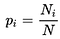Fig. 7. Defining p_i for a system of particles

Here, N_i is the number of particles that were found to be in the state i, while N is the total number of particles in the system. If we use the Boltzmann distribution to explain this probability we end up with: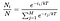Fig. 8. Boltzmann distribution for statistical mechanics

This turns out to be an accurate assessment of energy states, and is explored in the field of spectroscopy. In an extremely reductive way, spectroscopy is the measurement of the emission or absorption of energy when light and matter interact. If we picture a system of atoms that could occupy 2 different states, when they transition from one to the other they must absorb or emit energy in the form of electromagnetic radiation. This energy is described as a spectral line, and if we observe a strong spectral line for a certain transition at a certain temperature, then there is likely a large fraction of atoms/molecules in the system that exist in the first state. This can be expanded to M number of states and helps scientists gauge how atoms behave under certain conditions individually, as well as systemically, thus bridging the gap between micro and macro scale systems.

Which brings us to the first question.

Back in the first section of this article we discussed that an isolated system spontaneously moves towards thermodynamic equilibrium, which is the state with maximum entropy. Well through a wonderful turn of events, the Boltzmann distribution is the distribution that maximizes the entropy. It also allows for us to find equivalence between the two definitions of entropy we started with: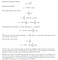Fig. 9. Derivation to explain equivalence of the two entropy formulae via the Boltzmann distribution

The above derivation shows that through the Boltzmann’s distribution we can satisfy the necessary conditions for equivalence between the quantum/statistical mechanics and thermodynamics definitions of entropy, bringing us full circle for our discussion of entropy in terms of this simple and powerful probability distribution.

## Boltzmann Distribution and Machine Learning

Back in Fig. 6 we defined what the generalized form of the Boltzmann distribution looks like. Through a fascinating twist of fate, the Boltzmann distribution has the same structure as the softmax function in machine learning.

Commonly used as the last activation function of a neural network, the softmax function normalizes a set of numbers into a set of probabilities that sum up to 1.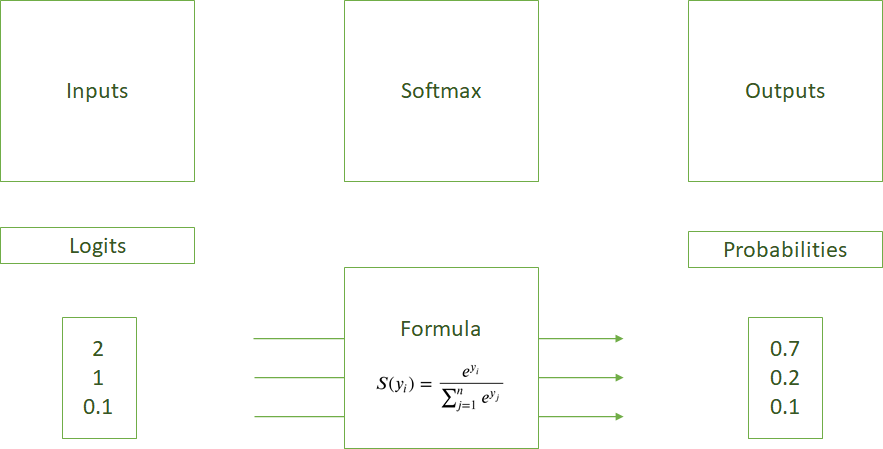Fig. 10. Schematic (based on this article) of how the Softmax function works

Here we apply the exponential function to each element of the input vector, and then normalize the value by dividing by the sum of these exponential values for all elements of the input vector. Since these probabilities sum up to 1 and the function itself employs exponential terms instead of normalizing the values directly, softmax helps models like neural networks converge more quickly to the correct form than they otherwise would.

That concludes this short overview of Boltzmann distribution and its impact on science, machine learning and entropy. In an increasingly chaotic universe, thank you for taking the time to read this article. There is beauty behind randomness.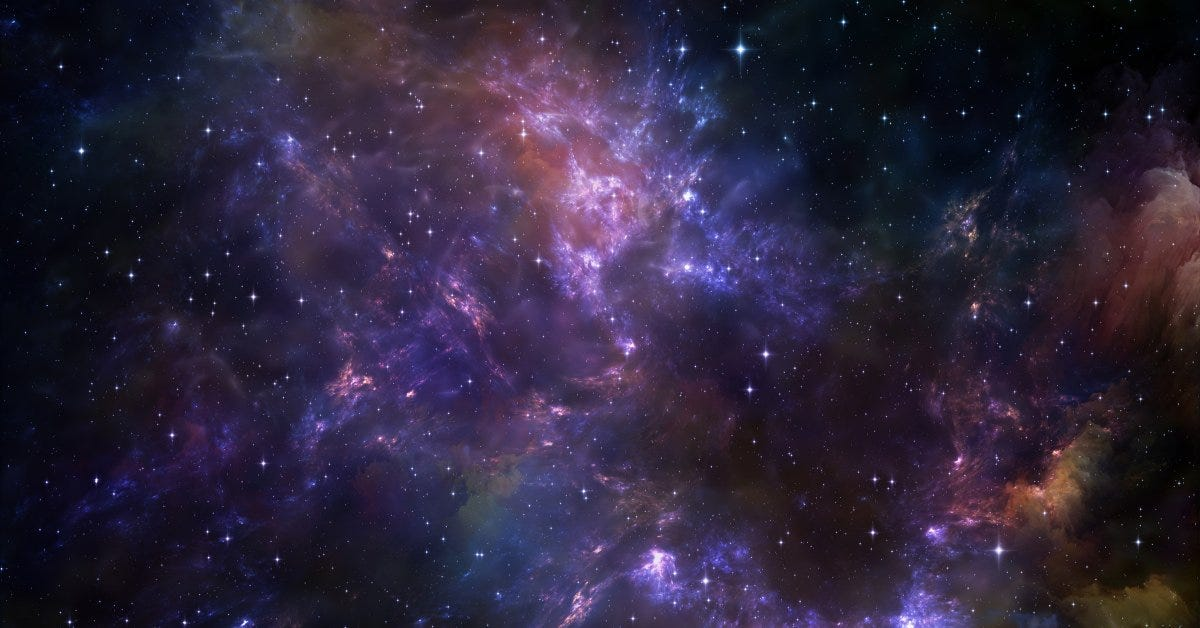©agsandrew (Getty Photos/iStockPhoto) via Time Magazine

Chemical, Biochemical and Environmental Engineering, Data Science